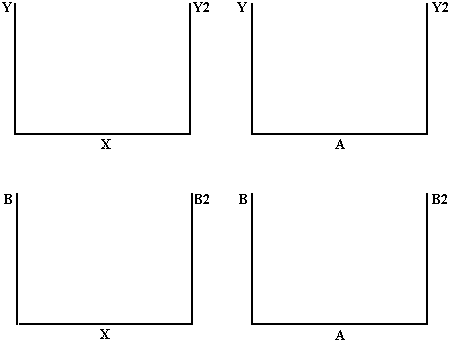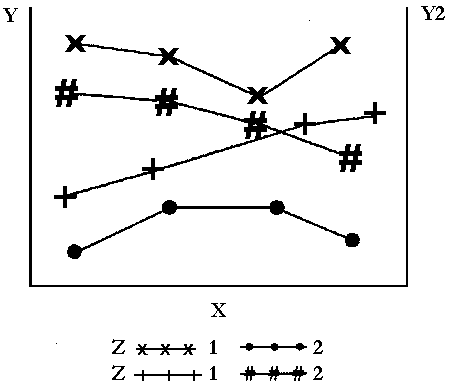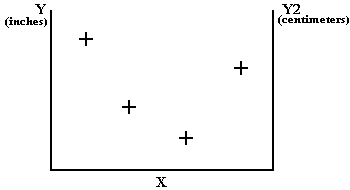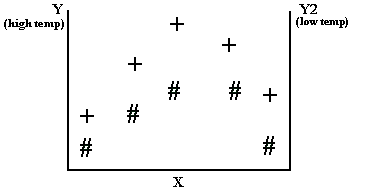The GPLOT Procedure

# PLOT2 Statement

Produces one or more plots with the vertical axis on the right side of the graph against which a second variable can be plotted.
 Requirements: You cannot use the PLOT2 statement alone. It can be used only with a PLOT or BUBBLE statement. At least one plot request is required. Global statements: AXIS, FOOTNOTE , LEGEND , PATTERN, SYMBOL, TITLE

### Description

The PLOT2 statement specifies one or more plot requests that name the horizontal and right vertical axis variables. This statement automatically does the following:

• plots data points within the axes

• scales the axes to include the maximum and minimum data values

• labels each axis with the name of its variable and displays each major tick mark value

You can use statement options to manipulate the axes and modify the appearance of your graph. You can use SYMBOL definitions to modify plot symbols for the data points, join data points, draw regression lines, plot confidence limits, or specify other types of interpolation. For more information on the SYMBOL statement see About SYMBOL Definitions.

Note:   When using the PLOT2 statement to generate output with the Java or ACTIVEX device drivers, and when the global statement SYMBOL is used, the value of the SYMBOL statement option INTERPOL= cannot be BOX, STD, or HILO.In addition, you can use global statements to modify the axes; to add titles, footnotes, and notes to the plot; or to modify the legend if one is generated by the plot. You can also use an Annotate data set to enhance the plot.

### Syntax

 PLOT2 plot-request(s) ;

option(s) can be one or more options from any or all of the following categories:

• plot options:

 AREAS=n GRID LEGEND | LEGEND=LEGEND<1...99> NOLEGEND OVERLAY REGEQN SKIPMISS
• appearance options:

 ANNOTATE=Annotate-data-set CAXIS=axis-color CFRAME=background-color COUTLINE=outline-color CTEXT=text-color FRAME | NOFRAME NOAXIS | NOAXES
• vertical axis options:

 AUTOVREF CAUTOVREF=reference-line-color CVREF=reference-line-color | (reference-line-color) | reference-line-color-list LAUTOVREF=reference-line-type LVREF=reference-line-type | (reference-line-type) | reference-line-type-list VAXIS=value-list | AXIS<1...99> VMINOR=n VREF=value-list VREVERSE VZERO
• ODS options:

 HTML=variable HTML_LEGEND=variable

plot-request(s)

each specifies the variables to plot and produces a separate graph, unless you specify the OVERLAY option. All variables must be in the input data set. Multiple plot requests are separated with blanks. A plot request can be any of these:

y-variable*x-variable<=n>

plots the values of two variables and can assign a SYMBOL definition to the plot.

y-variable

variable plotted on the right vertical axis.

x-variable

variable plotted on the horizontal axis.

n

number of the nth generated SYMBOL definition.

(y-variable(s))*(x-variable(s))

plots the values of two or more variable and produces a separate graph for each combination of Y and X variables.

y-variable(s)

variables plotted on the right vertical axes.

x-variable(s)

variables plotted on the horizontal axes.

y-variable*x-variable=third-variable

plots the values of two variables against a third classification variable

y-variable

variable plotted on the right vertical axis.

x-variable

variable plotted on the horizontal axis.

third-variable

classification variable against which y-variable and x-variable are plotted. Third-variable can be character or numeric, but numeric variables should contain discrete rather than continuous values, or should be formatted to provide discrete values.

In a PLOT2 plot request, the X variable for the horizontal axis must be the same as in the accompanying PLOT or BUBBLE statement. Typically, the Y variable for the right vertical axis is different.

Use the same types of plot requests with a PLOT2 statement that you use with a PLOT statement, but a PLOT2 statement always plots the values of y-variable on the right vertical axis.

Options for the PLOT2 statement are identical to the options for the PLOT statement except for these options, which are ignored if you specify them:

 AUTOHREF CAUTOHREF= CHREF= DESCRIPTION= HAXIS= HMINOR= HREF= HREVERSE= HZERO= IFRAME= IMAGESTYLE = LAUTOHREF= LHREF= NAME= WHREF= WAUTOHREF=

See PLOT Statement for descriptions of options that you can use with the PLOT2 statement.

The plot requests in both the PLOT and PLOT2 statements must be evenly matched as in this example:

```plot  y*x  b*a;
plot2 y2*x b2*a;```

These statements produce two graphs, each with two vertical axes. The first pair of plot requests (Y*X and Y2*X) produce one graph in which X is plotted on the horizontal axis, Y is plotted on the left axis, and Y2 is plotted on the right axis. The second pair of plot requests (B*A and B2*A) produce another graph in which A is plotted on the horizontal axis, B is plotted on the left axis, and B2 is plotted on the right axis.

### Using Multiple Plot Requests

Plot requests of the form (y-variable(s))*(x-variable(s)). Both the PLOT and PLOT2 statements generate multiple graphs (the actual plots are produced on separate pages). See Graphs Produced by Multiple Plot Requests in PLOT and PLOT2 Statements.

```plot (y b)*(x a);
plot2 (y2 b2)*(x a);```### Requesting Plots of Three Variables with a Legend

When both the PLOT and PLOT2 statements use plot requests of the form y-variable*x-variable=third-variable, each statement generates a separate legend. If the third variable has two values, these statements produce one graph with four sets of data points. See Multiple Plots on One Graph. The figure assumes that SYMBOL statements are used to specify the plot symbols that are shown and to connect the data points with straight lines.

```plot y*x=z;
plot2 y2*x=z;```### Displaying the Same Values in a Different Scale

If your data contain the same variable values in two different scales, such as height in inches and height in centimeters, you can display one scale of values on the left axis and the other scale of values on the right axis. If both vertical axes are calibrated so that they represent the same range of values, then for each observation of X the data points for Y and Y2 are the same.

For example, if Y is height in inches and Y2 is height in centimeters and if the Y axis values range from 0 to 84 inches and the Y2 axis values range from 0 to 213.36 centimeters, the plot is like Right Axis with Different Scale of Values.For these types of plots, the PLOT2 statement should use a SYMBOL statement that specifies INTERPOL=NONE and VALUE=NONE.

### Displaying Different Values

If your data contain variables with different data values (such as height and weight), you can display one type of data on the left axis and another type of data on the right axis. Because the Y variable and the Y2 variable contain different data, two sets of data points are displayed on the graph. For example, if Y is height and Y2 is weight, the plot is like Right Axis with Different Values and Different Scale.### Displaying the Same Scale on Both Axes

If your data contain two sets of values for the same type of data, you can use the PLOT2 statement to generate a right axis that is calibrated the same as the left axis so that the data points on the right of the graph are easier to read. For example, if Y is high temperatures and Y2 is low temperatures, you can create a graph like Right Axis with Same Scale of Values.To scale both axes the same, specify the same range of values either with the VAXIS= option in both the PLOT and PLOT2 statements, or with AXIS statements.

The PLOT2 statement uses PATTERN and SYMBOL definitions in the same way the PLOT statement does. These definitions are assigned in order first to the PLOT statement and then to the PLOT2 statement.